# 3D Formulas

These are also known as cubed formulas.
A reference that refers to the same cell or range on multiple worksheets is called a 3D reference.
Using 3D formulas allows you to calculate data throughout a workbook using multiple worksheets.
All 3D formulas are based on the syntax: Sheet1:Sheet4!A2:B5.
A 3D formula is a formula that refers to the same cell (or range of cells) on multiple worksheets.
The 3D formula "=SUM(Sheet1:Sheet4!A2)" can be used to add up the numbers in cell "A2" on 4 different worksheets.
If you copy or insert a new worksheet after Sheet1 the reference will automatically include it.
Similarly if you delete a worksheet it will be excluded.

If you copy or insert a new worksheet after Sheet1 the reference will automatically include it.
Similarly if you delete a worksheet it will be excluded.

Lets assume that we have a workbook that contains five worksheets and that four of them contain data for specific years.
Four of the worksheets correspond to the sales figures for the years 2005 - 2002 and the first worksheet is intended to be a summary of these four years.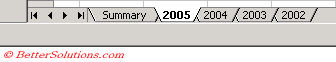On the Summary worksheet we want to be able to quickly return the total for all the Regions and for the months.
It is possible to create 3D formulas which refer to all four worksheets which makes creating a summary worksheet very easy.
Lets assume that each of the four worksheets contains the following table of data.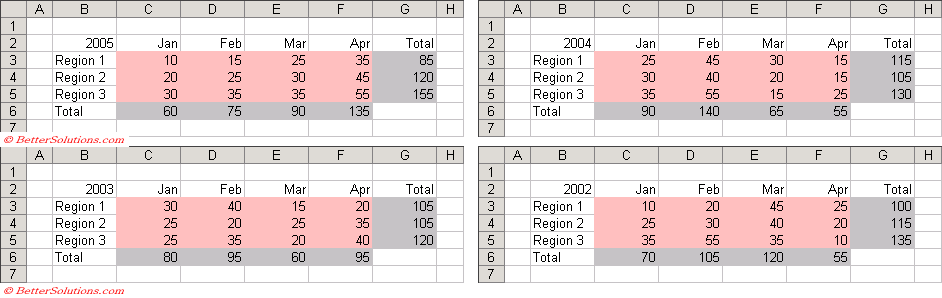### Inserting the Formula

We are going to insert a 3D formula for each of the items we want to total.
The first item in our summary table is the total for Region 1.
Select cell "C2" and insert the SUM function as normal.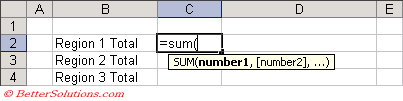Select the "2005" worksheet tab with the mouse.
Hold down the Shift key and select the "2002" worksheet tab with the mouse.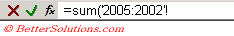Select the cell you want to sum in this case cell "G3".
Enter a close bracket ")" to complete the formula.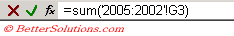Create the following table on the Summary worksheet.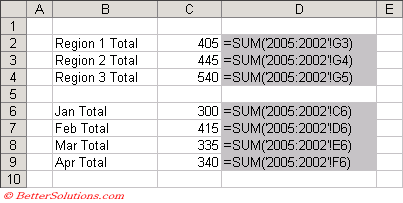Repeat the above steps for the other six totals to create your summary worksheet.

### Worksheet Functions

It is important to realise that not all the functions will accept a 3D formula.
The following functions can all be used in 3D formulas.

 AND The logical AND for any number of arguments. AVEDEV The average deviation for a list of numbers. AVERAGE The arithmetic mean of a list or array of numbers. AVERAGEA The arithmetic mean of a list or array of numbers, including text and logical values. COUNT The number of cells with a numeric value in a list or cell range. COUNTA The number of non blank cells in a list or cell range. DEVSQ The sum of squares of deviations of data points from their sample mean. LARGE The Kth largest value in an array of numbers. MAX The largest value in a list or array of numbers. MAXA The largest value in a list or array of numbers, including text and logical values. MEDIAN The median of the numbers in a list or cell range. MIN The smallest number in a list or range. MINA The smallest number in a list or range, including text and logical values OR The logical OR for any number of arguments. PERCENTILE The Kth percentile of values in a range. PRODUCT The product of all the numbers in a list or cell range. QUARTILE The quartile of a data set. RANK The rank of a value in a range (in descending order). SKEW The number representing the skewness of a distribution. SMALL The Kth smallest value in an array of numbers. STDEV The standard deviation based on a sample. STDEVA The standard deviation based on a sample, including text and logical values. STDEVP The standard deviation based on an entire population. STDEVPA The standard deviation based on an entire population, including text and logical values. SUM The total value of the numbers in a list or cell range. SUMSQ The sum of the squares of all the values in a list or cell range. TRIM The text string with all spaces removed from the beginning and end. VAR The compound variance based upon the numerical values in the range. VARA The compound variance based upon the numerical values in the range. VARP The variance based on an entire population. VARPA The variance based on an entire population, including text and logical values.

### Important

It is possible to create named ranges for 3D cell references which can be used to simplify your 3D formulas.